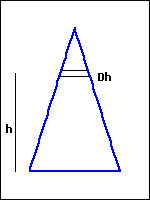Consider the area shown below. The height of the triangle is 5 and the length of its base is 3. We have used the notation $Dh$ for $\Delta h$.Write a Riemann sum for the area, using the strip shown and the variable $h$:
Riemann sum $= \Sigma$

Now write the integral that gives this area:
area $= \int_a^b\,$
where $a =$
and $b =$

Finally, calculate the exact area of the region, using your integral.
area =

You can earn partial credit on this problem.